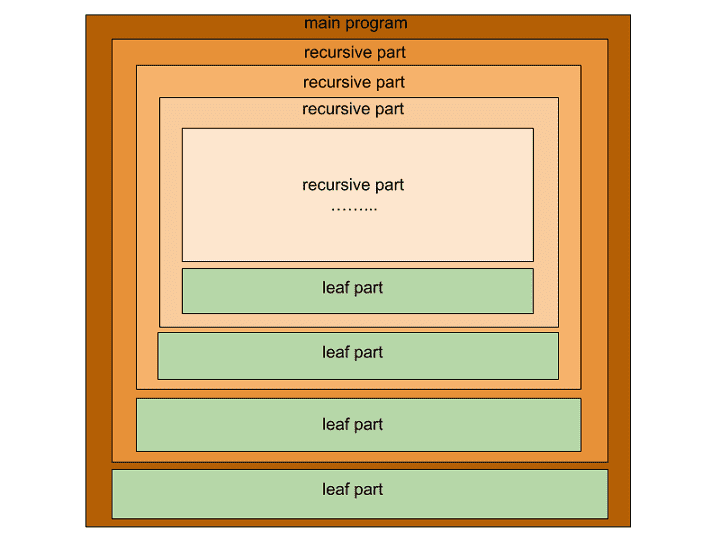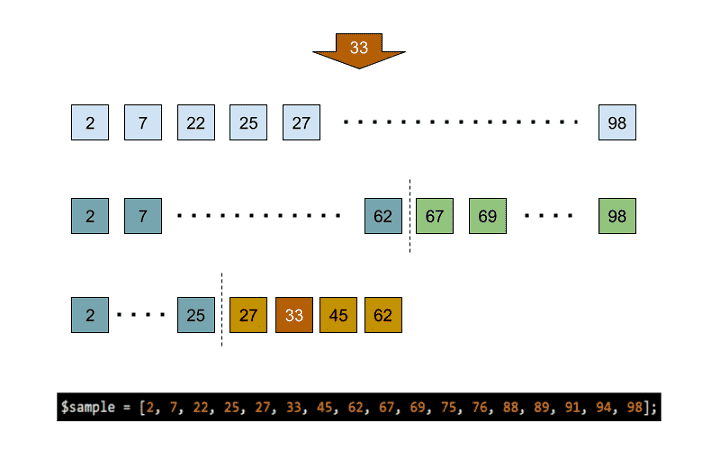> > Loop Multidimensional Array in PHP by 3 Recursive Demos

# Loop Multidimensional Array in PHP by 3 Recursive Demos

You can walk across one-dimensional array simply, but may laboriously loop through multidimensional array in PHP using recursive methods. Truly, the multidimensional features in data need more efforts for traversal of array.

Obviously, recursive methods involve many types of applications such as traversing files in a directory, Towers of Hanoi, types of Tree Traversal and so on.

All codes here are not complicated, so you can easily understand even though you are still students in school. To benefit your learning, we will provide you download link to a zip file thus you can get all source codes for future usage.

We have released it under the MIT license, so feel free to use it in your own project or your school homework.

• Prepare HTTP server such as XAMPP or WAMP in your windows environment.
• Download and unzip into a folder that http server can access.

## DEMO 1Traverse Values Only

What is recursive method? We concisely introduce it in the section. The first demo walks PHP array in recursive method and display each item by values only, not including index.

### Recursive Method

A recursive method is an approach for problem solving that divides a problem into smaller varieties of itself. When solving all subproblems entirely, the solution to the major problem is thus present.

In general, a recursive program consists of recursive parts and leaf parts. The recursive parts contain calls to itself, but the leaf parts are normal sequential programming steps. A recursive solving process seems to gather result of leaf parts, and contribute to a final answer gradually layer by layer in an upward direction.

Conceptually, a recursive part is divided into a child recursive part and a leaf part. And the recursive process goes on iteratively in each layer. As the picture illustrates below.In computer science, the recursive approach can be applied to many types of problems such as walking through all files in a folder, algorithm for Towers of Hanoi and so on. In the article, we demonstrate it by the examples that loop multidimensional array in PHP.

### Loop Multidimensional Array in PHP

First, we should have an array containing multilayer of data. Using PHP function `print_r()` helps us to see the array structure.

recursive.php
``````// Array for traversal
\$sample = ['vehicle'=>'car', 'animal'=>['dog', 'goat'], 'food'=>['egg', 'fruit'=>['apple', 'banana'], 'vegetable', 'cake'], 'planet'=>['Mars', 'Jupiter', 'Venus'], 'color'=>'blue'];
``````
```Array
(
[vehicle] => car
[animal] => Array
(
 => dog
 => goat
)
[food] => Array
(
 => egg
[fruit] => Array
(
 => apple
 => banana
)
 => vegetable
 => cake
)
[planet] => Array
(
 => Mars
 => Jupiter
 => Venus
)
[color] => blue
)
```

As mentioned previously, there are a recursive part and a leaf part in the PHP scripts. And the programs below use such a rule to divide tasks. If the first argument is an array, a recursive part starts its tasks. However, for the leaf part, just print out values along with extra indents of different length according to layers.

recursive.php
``````// 1st Demo
function recursive_1(\$value, \$indent=-1) {
if (is_array(\$value)) {
echo "\n";
foreach(\$value as \$v) {
recursive_1(\$v, \$indent+1);
}
} else {
}
}
echo "\n******* recursive demo 1 *******\n\n";
recursive_1(\$sample);
``````

From the traversal result, the 1st layer has car and blue. The 2nd layer has dog, goat, egg, vegetable, cake, Mars, Jupiter, and Venus. At bottom, the 3rd layer has apple and banana.

```******* recursive demo 1 *******
car
dog
goat
egg
apple
banana
vegetable
cake
Mars
Jupiter
Venus
blue
```

## DEMO 2Traverse Keys and Values

Based on the concept mentioned before, let us walk across PHP array and show both keys and values by more advanced recursive approach. That is the 2nd demo.

### Loop Multidimensional Array in PHP for Keys and Values

This version of recursive program traverses not only values, but also keys. One more argument for keys is added in the 2nd position. This additional argument has empty value as default that ignores printing key values in the first layer.

recursive.php
``````// 2nd Demo
function recursive_2(\$value, \$key="", \$indent=-1) {
if (is_array(\$value)) {
if(\$key!="") {
}
foreach(\$value as \$k=>\$v) {
recursive_2(\$v, \$k, \$indent+1);
}
} else {
}
}
echo "\n******* recursive demo 2 *******\n\n";
recursive_2(\$sample);
``````

The result for this version is below. It contains keys such as vehicle for car, and animal for dog and goat.

```******* recursive demo 2 *******
vehicle => car
animal =>
0 => dog
1 => goat
food =>
0 => egg
fruit =>
0 => apple
1 => banana
1 => vegetable
2 => cake
planet =>
0 => Mars
1 => Jupiter
2 => Venus
color => blue
```

## DEMO 3PHP Built-In Function

Also, PHP provides built-in function to loop multidimensional array in PHP. The 3rd demo indicates the pros and cons of applying such a method. Indeed, it has disadvantages.

### PHP Build-In array_walk_recursive()

Alternatively, to loop multidimensional array in PHP using built-in functions seems a effortless method, but the result may not satisfy you.

The PHP build-in function `array_walk_recursive()` requires a function pointer as the 2nd argument. In fact, it quickly traverses all keys and values, but prints only leaf nodes information. The result will not represent data structure that contains intermediate keys.

recursive.php
``````// 3rd Demo
function recursive_3(\$value) {
array_walk_recursive(\$value, "show_data");
}
function show_data(\$value, \$key) {
echo "\$key => \$value\n";
}
echo "\n******* recursive demo 3 *******\n\n";
recursive_3(\$sample);
``````

To loop multidimensional array in PHP in this version generates output as below. Unfortunately, it is too simple to show complete information for array data structure.

```******* recursive demo 3 *******
vehicle => car
0 => dog
1 => goat
0 => egg
0 => apple
1 => banana
1 => vegetable
2 => cake
0 => Mars
1 => Jupiter
2 => Venus
color => blue
```

## DEMO 4More Recursive Examples

In addition, we have two more useful examples using recursive method, even though they has nothing to do with looping multidimensional array in PHP.

### Walk Through Files in a Folder

The application is common and useful. Moreover, it is difficult and even impossible to traverse a folder without using recursive approaches. The example walks a PHP array in recursive method, For each item found, actions are to print out and to check whether the item is a directory. If true, call itself to execute the codes of recursive part.

traverse-folder.php
``````function list_files_in_folder(\$dir){
\$ffs = scandir(\$dir);

unset(\$ffs[array_search('.', \$ffs, true)]);
unset(\$ffs[array_search('..', \$ffs, true)]);

foreach(\$ffs as \$ff){
echo "\$dir/\$ff\n";
if(is_dir("\$dir/\$ff")) list_files_in_folder("\$dir/\$ff");
}
}

echo "\n******* Traverse folder *******\n\n";
list_files_in_folder("test-folder");
``````

For example, we make a dummy folder for testing that has structure like the following. Of course, `list_files_in_folder()` will output the same result.

```c:\> php traverse-folder.php
******* Traverse folder *******
test-folder/test-1
test-folder/test-1/test-1a
test-folder/test-1/test-1b
test-folder/test-2
test-folder/test-2/test-2a
test-folder/test-2/test-2b
test-folder/test-2/test-2c
test-folder/test-3
test-folder/test-3/test-31
test-folder/test-3/test-31/test-31a
test-folder/test-3/test-3a
test-folder/test-a
test-folder/test-b
```

### Binary Search

The binary search algorithm is famous, so we give a recursive version here to discuss with you. According its rules, the sorted array data is repeatedly divided into two sub-arrays evenly, but only one of two recursive parts is executed each time. The target value to search for is 33.binary-search.php
``````function binary_search(\$list, \$begin, \$end, \$target){

\$middle = floor( (\$begin + \$end)/2 );
if (\$list[\$middle] == \$target) {
return "sample[\$middle] => \$target\n";
}
elseif (\$target < \$list[\$middle]) {
// recursive call itself on [begin, middle - 1]
return binary_search(\$list, \$begin, \$middle - 1, \$target);
}
else {
// recursive call itself on [middle + 1, end]
return binary_search(\$list, \$middle + 1, \$end, \$target);
}
}

// main
echo "\n******* Binary search *******\n\n";
\$sample = [2, 7, 22, 25, 27, 33, 45, 62, 67, 69, 75, 76, 88, 89, 91, 94, 98];
\$target = (\$argc > 1) ? \$argv : '7';

echo binary_search(\$sample, 0, count(\$sample), \$target);
``````

Until the function `binary_search()` decides that the target value is found or that it is not found, actions are to print the location of target value or to show message of “not found”. Operationally, we uses PHP argument variable `\$argv` to get a target value to search for.

```c:\> php binary-search.php 7
******* Binary search *******
sample => 7

c:\> php binary-search.php 67
******* Binary search *******
sample => 67

c:\> php binary-search.php 94
******* Binary search *******
sample => 94

c:\> php binary-search.php 85
******* Binary search *******